# Modal interaction

The following shows an examplel of modal interactions for a 20 kHz $$\phi$$40 mm horn ($$c_{tw}$$ = 5100 m/sec; Poisson's ratio = 0.33). The horn length was tuned as needed to maintain the axial resonance (the maximum adjustment was 0.1 mm). Also see Amplitude asymmetry.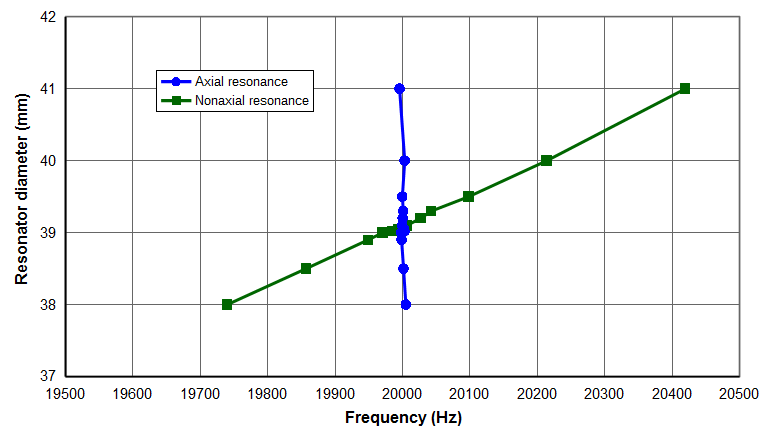Figure 1. Frequencies for a 20 kHz $$\phi$$40 mm hornFigure 2. Face amplitude asymmetries for a 20 kHz $$\phi$$40 mm horn

The following table shows some data from the above graphs. Note how quickly the axial mode asymmetry changes for small changes in the horn diameter and as the axial and nonaxial resonances converge. Also note how the nonaxial modeshape changes as it interacts with the axial mode.

Table notes —

1. All displacements (as represented by the image colors) are in the axial direction.
2. All displacements are displayed at the same scale.

Diameter (mm) Frequency
separation (Hz)
Axial mode Nonaxial mode
38.00 -265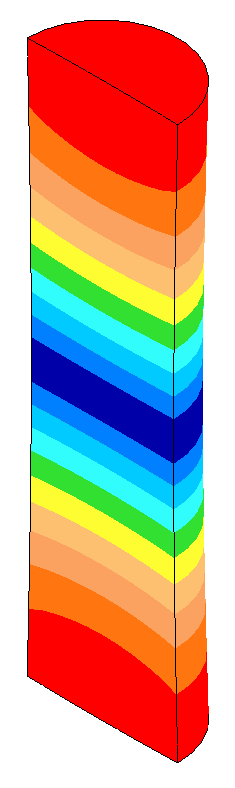20005 Hz Asymmetry = 0.001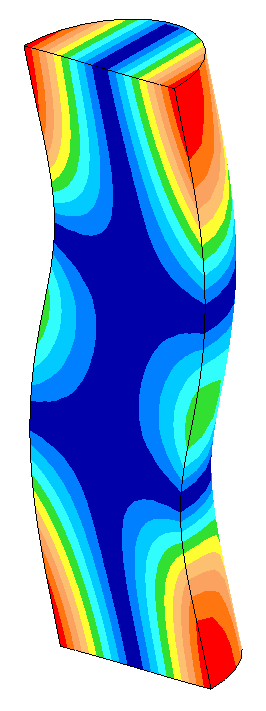19740 Hz
39.00 -27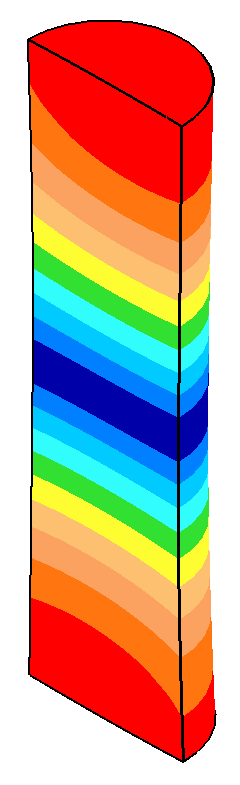20000 Hz Asymmetry = 0.06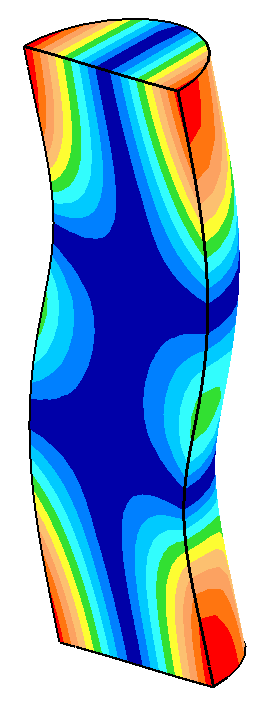19973 Hz
39.02 -18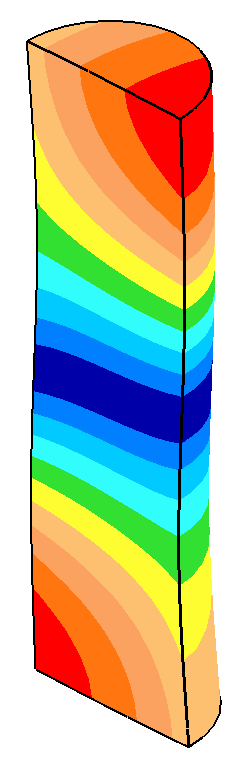19998 Hz Asymmetry = 0.34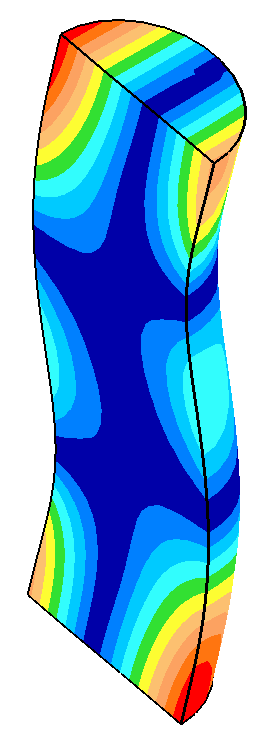19982 Hz
39.05 -12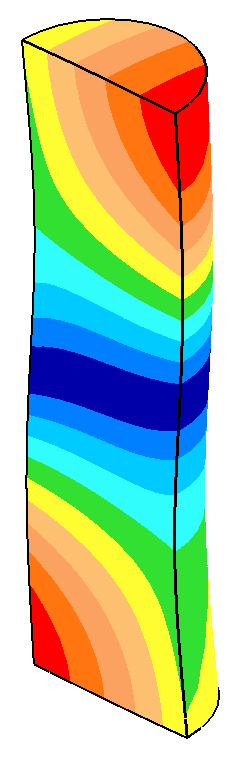20000 Hz Asymmetry = 0.50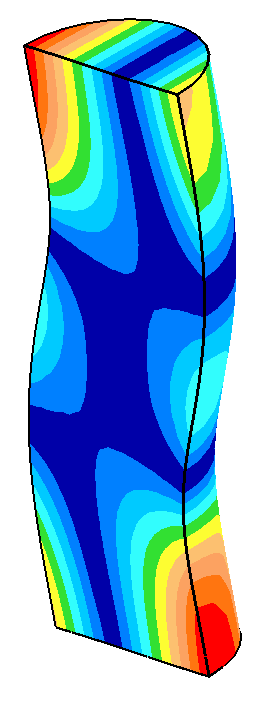19988 Hz
39.10 620000 Hz Asymmetry = 0.9620006 Hz
39.20 2720000 Hz Asymmetry = 0.18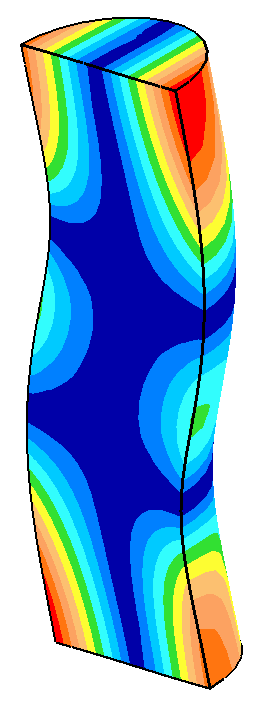20027 Hz
39.30 4220001 Hz Asymmetry = 0.06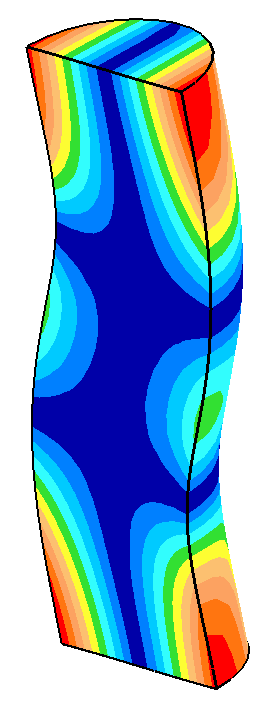20043 Hz
41.00 424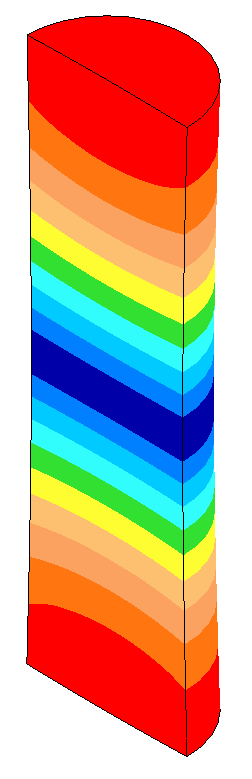19996 Hz Asymmetry = 0.008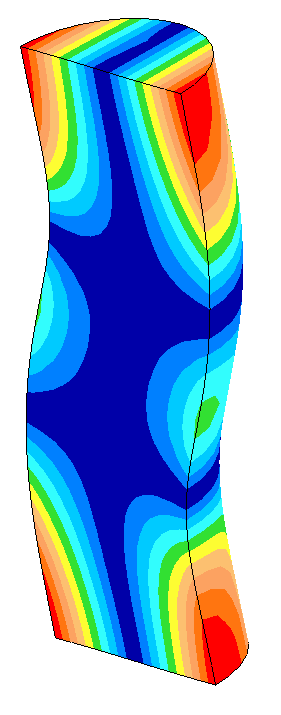20420 Hz